1. 清华大学 电机工程与应用电子技术系, 北京 100084;
2. 北京交通大学 电气工程学院, 北京 100044

Analysis of the high-frequency oscillation characteristics of a multi-port converter with an AC link
WEI Shusheng1, ZHAO Zhengming1, WEN Wusong1, LI Kai2, YUAN Liqiang1, CAI Weiqian1
1. Department of Electrical Engineering, Tsinghua University, Beijing 100084, China;
2. School of Electrical Engineering, Beijing Jiaotong University, Beijing 100044, China
Abstract: High-frequency links based on modular multi-active bridges (MMAB) are useful for high power multi-port converters since they are modular, efficient and isolate the various ports. High-frequency AC buses have intrinsic oscillations that do not occur in DC buses which increase the converter losses and make the system less safe. Also, oscillations in one module affect the operations of other modules connected to the common AC bus. This study modeled two kinds of converters with each having two modules to study the effects of the high-frequency oscillations in the AC-link in an MMAB. The frequency domain analysis method was then used to investigate the relationship between the oscillations and the resonant tank parameters, including the stray parameters of the high-frequency transformer (HFT) and the stray capacitance of the switching devices. The results showed the key factors leading to the oscillations for both the uncontrolled mode and the controlled mode. Tests were conducted for the two modes and for switching between the two modes. The analytical results are verified by experimental data which would lay a foundation to reduce the oscillations in MMAB in the future.
Key words: AC link bus    modular multi-active bridge (MMAB)    high-frequency oscillation    stray parameters

1 共高频交流母线变换器 1.1 共交流母线拓扑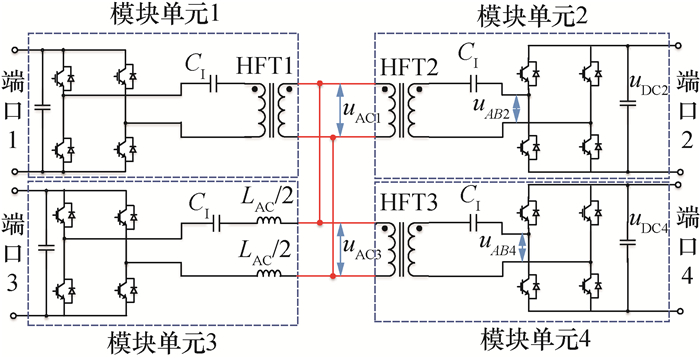图 1 共交流母线拓扑电能路由器结构

1.2 主要工作模式

1) 变换器内部多模块的启动过程。多模块变换器中，子模块的控制系统往往是从各自的直流母线取电，在直流母线电压建立起来之前，控制电路和驱动电路不工作，开关管不受控。与传统DAB的预充电过程类似，采用一个端口的模块通过高频交流母线对剩余模块充电，整个过程被充电单元开关管闭锁。

2) 端口主动待机或关闭。在正常工作时，对多端口变换器，某些端口不需要工作或处于待机状态时，该端口对应模块开关管闭锁。

3) 变换器故障运行。对于多端口变换器，当某个端口发生故障时，其余正常端口维持工作，此时，系统处于不控整流模式。图 2 (网络版彩图)启动过程振荡电压波形

2 两模块共高频交流母线变换器分析 2.1 两模块单元组成基本变换器结构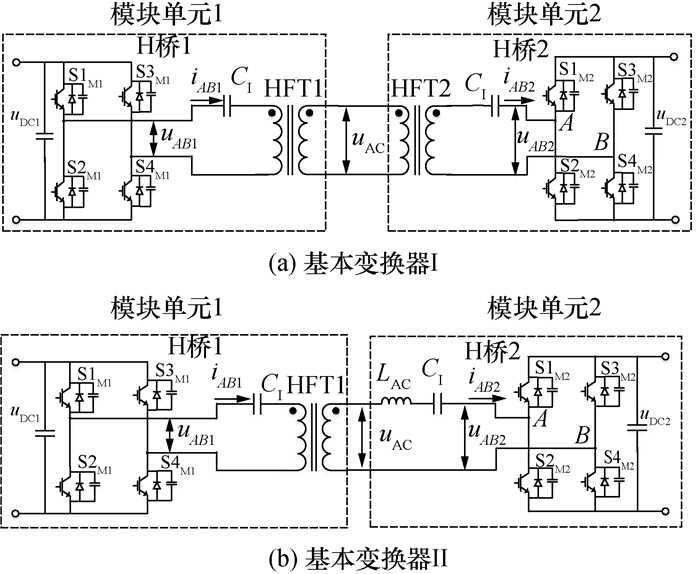图 3 两模块并联共交流母线拓扑

2.2 变压器和电感等效模型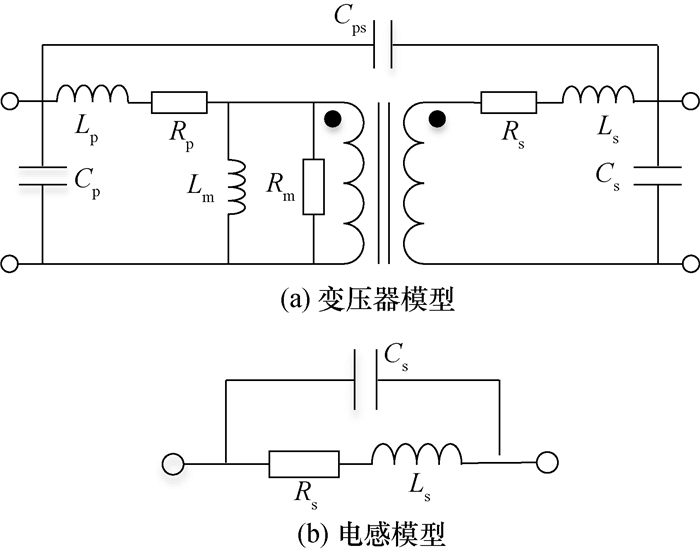图 4 考虑杂散参数的变压器和电感模型

2.3 开关器件的杂散电容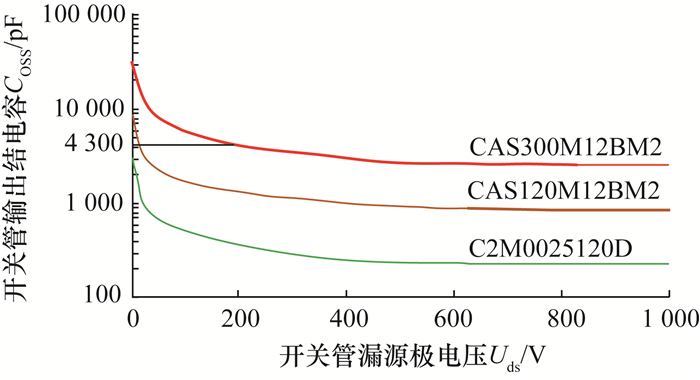图 5 (网络版彩图)SiC MOSFET输出结电容曲线

2.4 高频母线的杂散参数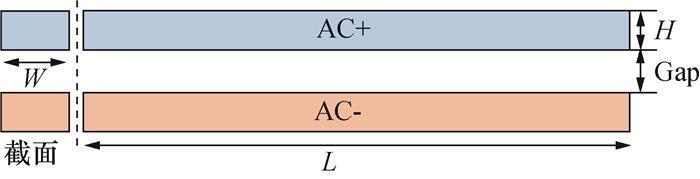图 6 交流母排结构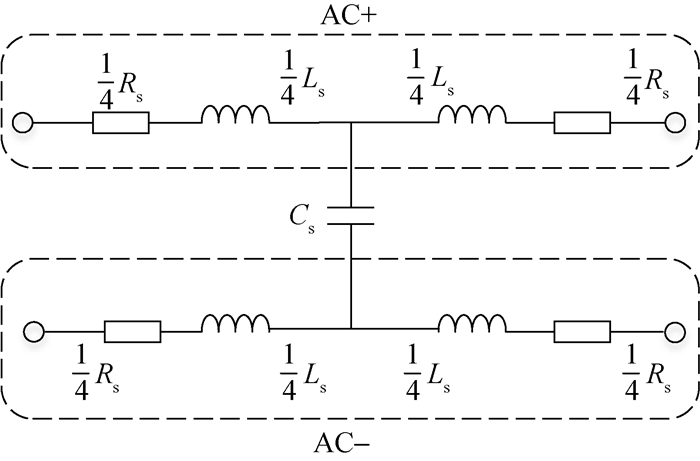图 7 正负母排等效杂散电路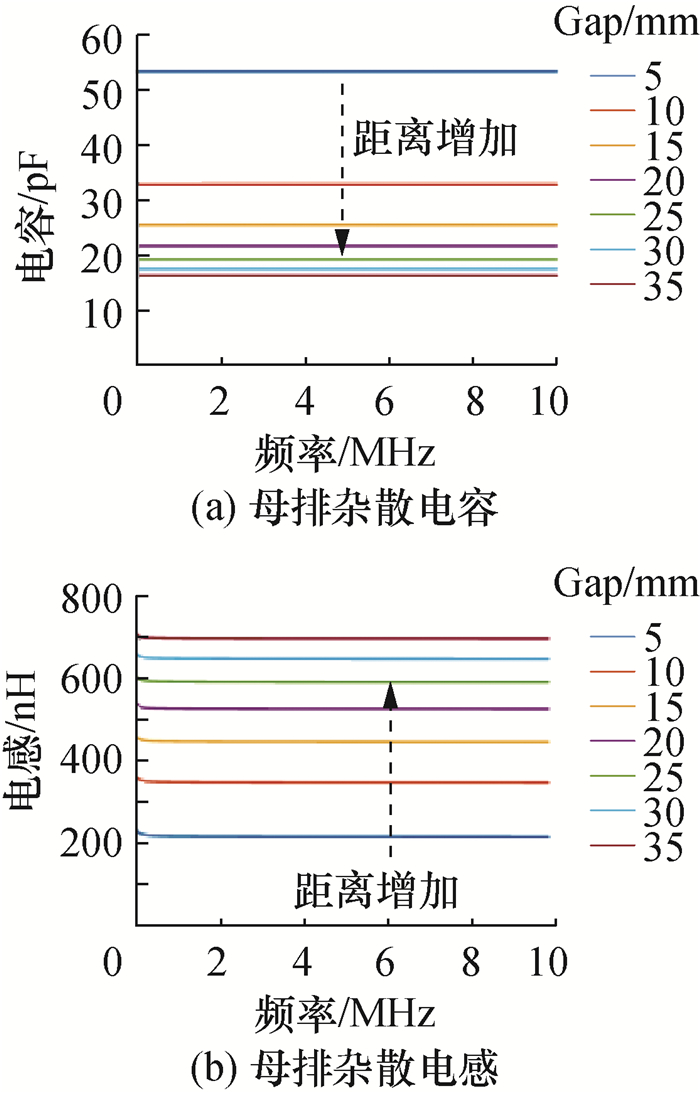图 8 (网络版彩图)母排杂散参数

3 不控整流模式

3.1 电路分析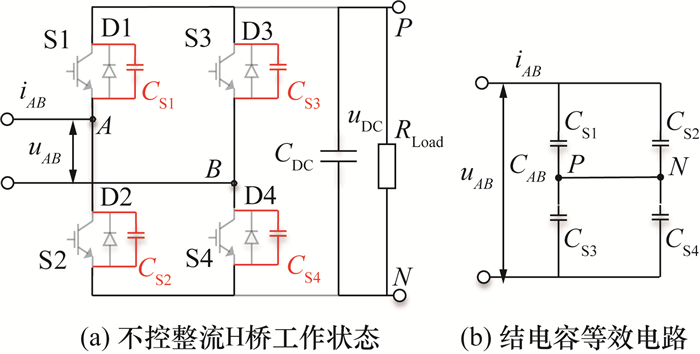图 9 不控整流模式下单元2工作状态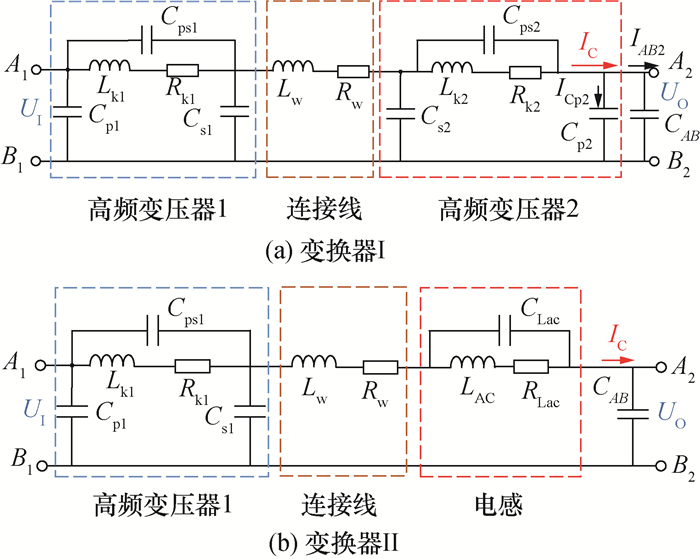图 10 不控整流模式下变换器的高频阻抗网络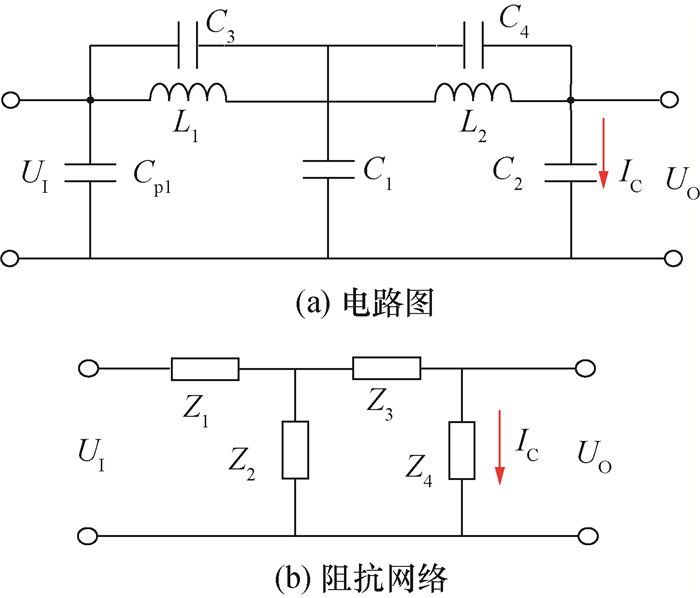图 11 不控整流模式阻抗网络

3.2 阻抗网络特性分析

 ${I_{\rm{C}}} = \frac{{{Z_2}{U_1}}}{{{Z_1}{Z_2} + {Z_1}{Z_3} + {Z_1}{Z_4} + {Z_2}{Z_3} + {Z_2}{Z_4}}}.$ (1)

 ${Y_{\rm{C}}} = \frac{{{I_{\rm{C}}}}}{{{U_{\rm{I}}}}} = \frac{{{Z_2}}}{{{Z_1}{Z_2} + {Z_1}{Z_3} + {Z_1}{Z_4} + {Z_2}{Z_3} + {Z_2}{Z_4}}}.$ (2)

 ${Y_{\rm{C}}}(s) = \frac{{{m_1}{\kern 1pt} {s^5} + {m_2}{\kern 1pt} {s^3} + {m_3}{\kern 1pt} s}}{{{n_1}{\kern 1pt} {s^4} + {n_2}{\kern 1pt} {s^2} + {n_3}}}.$ (3)

 $\left\{ {\begin{array}{*{20}{l}} {{m_1} = {L_1}{L_2}{C_2}{C_3}{C_4},}\\ {{m_2} = {C_2}({L_2}{C_4} + {L_1}{C_3}),}\\ {{m_3} = {C_2},}\\ {{n_1} = {L_1}{L_2}{C_2}{C_3} + {L_1}{L_2}{C_3}{C_4} + {L_1}{L_2}{C_2}{C_4} + }\\ {\quad {L_1}{L_2}{C_1}{C_2} + {L_1}{L_2}{C_1}{C_4},}\\ {{n_2} = {L_2}{C_2} + {L_2}{C_4} + {L_1}{C_3} + {L_1}{C_2} + {L_1}{C_1},}\\ {{n_3} = 1.} \end{array}} \right.$ (4)

 $\left\{ {\begin{array}{*{20}{l}} {{s_{{\rm{p1,2}}}} = \pm \sqrt {\frac{{{n_2} - \sqrt {n_2^2 - 4{n_1}{\kern 1pt} {n_3}} }}{{2{n_1}}}} {\rm{j}},}\\ {{s_{{\rm{p3,4}}}} = \pm \sqrt {\frac{{{n_2} + \sqrt {n_2^2 - 4{n_1}{\kern 1pt} {n_3}} }}{{2{n_1}}}} {\rm{j}},}\\ {{s_{{\rm{z1}}}} = 0,}\\ {{s_{{\rm{z2,3}}}} = \pm \sqrt {\frac{1}{{{L_2}{C_4}}}} {\rm{j}},}\\ {{s_{{\rm{z4,5}}}} = \pm \sqrt {\frac{1}{{{L_1}{C_3}}}} {\rm{j}}.} \end{array}} \right.$ (5)

 参数 变换器Ⅰ 变换器Ⅱ L1 变压器1漏感：Lk1 变压器1漏感：Lk1 L2 变压器2漏感：Lk2 滤波电感：LAC C1 变压器1副边和变压器2副边电容之和：Cs1+ Cs2 变压器1副边电容：Cs1 C2 变压器2原边电容和模块单元2的H桥等效电容之和：Cp2+ CAB 模块单元2的H桥等效电容：CAB C3 变压器1原副边电容：Cps1 变压器1原副边电容：Cps1 C4 变压器2原副边电容：Cps2 交流电感杂散电容：CLac

 $\left\{ {\begin{array}{*{20}{l}} {{\omega _{{\rm{z1}}}} = 0,}\\ {{\omega _{{\rm{z2}}}} = \sqrt {\frac{1}{{{L_2}{C_4}}}} ,}\\ {{\omega _{{\rm{z3}}}} = \sqrt {\frac{1}{{{L_1}{C_3}}}} ,} \end{array}} \right.$ (6)

 $\left\{ {\begin{array}{*{20}{l}} {{\omega _{{\rm{p1}}}} = \sqrt {\frac{{{n_2} - \sqrt {n_2^2 - 4{n_1}{\kern 1pt} {n_3}} }}{{2{n_1}}}} ,}\\ {{\omega _{{\rm{p2}}}} = \sqrt {\frac{{{n_2} + \sqrt {n_2^2 - 4{n_1}{\kern 1pt} {n_3}} }}{{2{n_1}}}} .} \end{array}} \right.$ (7)

3.3 系统参数对极点分布的影响

 物理量 参数符号 数值 匝数比 N1:N2 1:1 原边漏感 Lp 8.5 μH 副边漏感 Ls 8.5 μH 原边电阻 Rp 15.5 mΩ 副边电阻 Rs 15.5 mΩ 原边绕组电容 Cp 269 pF 副边绕组电容 Cs 271 pF 原副边电容 Cps 52 pF 激磁电感 Lm 1.89 mH 激磁电抗 Rm 10 kΩ

 物理量 参数符号 数值 电感 LAC 14 μH 电感杂散电容 CLac 50 pF图 12 (网络版彩图)不控整流模式阻抗网络导纳波特图

 $\left\{ {\begin{array}{*{20}{l}} {{f_{{\rm{p1,I}}}} = 0.397{\kern 1pt} {\kern 1pt} {\kern 1pt} {\rm{MHz}},}\\ {{f_{{\rm{p2,I}}}} = 1.73{\kern 1pt} {\kern 1pt} {\kern 1pt} {\rm{MHz}},}\\ {{f_{{\rm{p1,II}}}} = 0.441{\kern 1pt} {\kern 1pt} {\kern 1pt} {\rm{MHz}},}\\ {{f_{{\rm{p2,II}}}} = 2.44{\kern 1pt} {\kern 1pt} {\kern 1pt} {\rm{MHz}},} \end{array}} \right.$ (8)

 $\left\{ {\begin{array}{*{20}{l}} {{T_{\rm{I}}} = 2.52{\kern 1pt} {\kern 1pt} {\kern 1pt} \mu {\rm{s}},}\\ {{T_{{\rm{II}}}} = 2.27{\kern 1pt} {\kern 1pt} {\kern 1pt} \mu {\rm{s}}.} \end{array}} \right.$ (9)

 $\left\{ {\begin{array}{*{20}{l}} {{f_{{\rm{LC,I}}}} = \frac{1}{{2\pi \sqrt {({L_{{\rm{k1}}}} + {L_{{\rm{k2}}}}){C_{{\rm{OSS}}}}} }} = 0.416{\kern 1pt} {\kern 1pt} {\kern 1pt} {\rm{MHz}},}\\ {{f_{{\rm{LC,II}}}} = \frac{1}{{2\pi \sqrt {({L_{{\rm{k1}}}} + {L_{{\rm{k2}}}}){C_{{\rm{OSS}}}}} }} = 0.436{\kern 1pt} {\kern 1pt} {\kern 1pt} {\rm{MHz}}.} \end{array}} \right.$ (10)

fLC, IfLC, II差异是由于变压器和电感值差异造成的。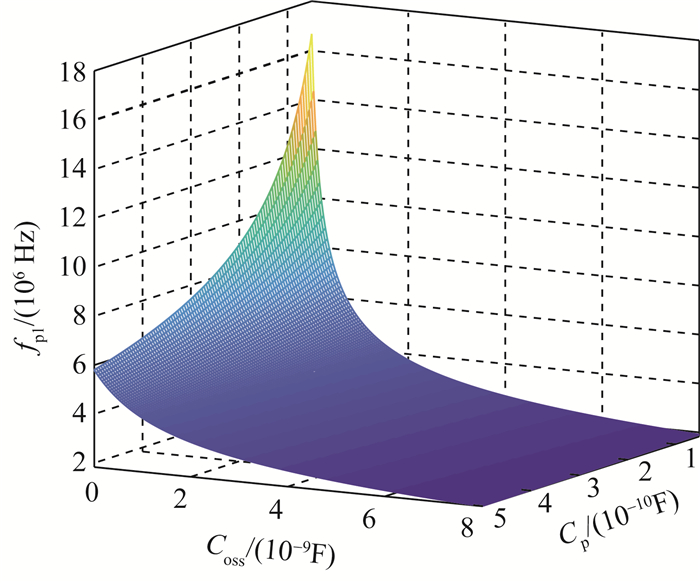图 13 谐振频率fp1和结电容COSS变压器杂散电容Cp的关系图 14 极点频率比fp2/fp1与电容值比COSS/Cp的关系

4 全受控模式 4.1 电路分析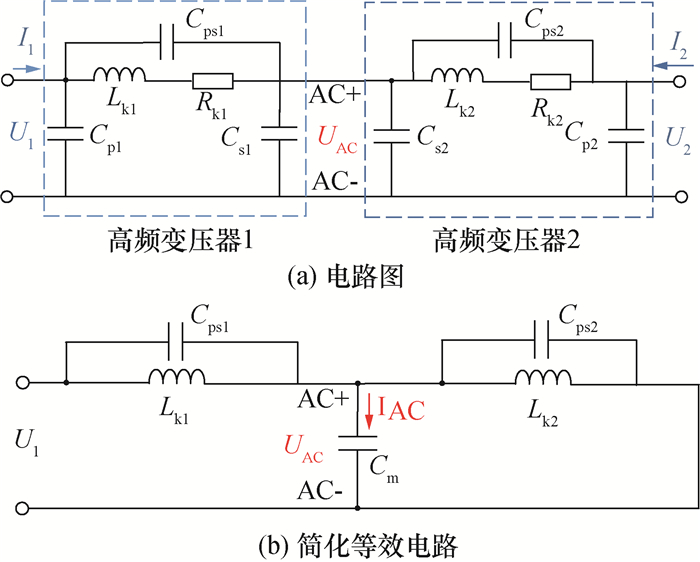图 15 变换器Ⅰ等效电路

4.2 阻抗网络特性分析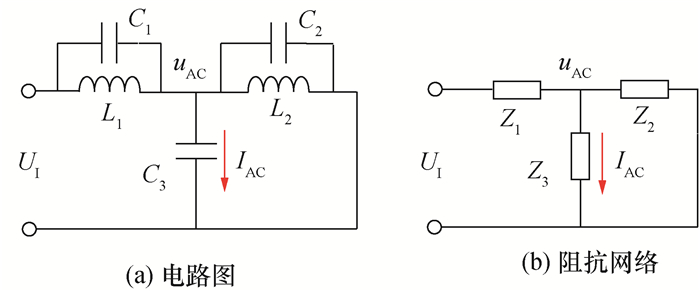图 16 全受控模式阻抗网络

 参数 变换器Ⅰ 变换器Ⅱ L1 变压器1漏感：Lk1 变压器1漏感：Lk1 L2 变压器2漏感：Lk2 滤波电感：LAC C1 变压器1原副边电容: Cps1 变压器1原副边电容: Cps1 C2 变压器2原副边电容：Cps2 滤波电感杂散电容：CLac C3 变压器1副边和变压器2副边电容之和：Cs1+ Cs2 变压器1副边电容：Cs1

 ${I_{{\rm{AC}}}} = \frac{{{Z_2}{U_1}}}{{{Z_1}{Z_2} + {Z_1}{Z_3} + {Z_2}{Z_3}}}.$ (11)

 $Y = \frac{{{I_{{\rm{AC}}}}}}{{{U_{\rm{I}}}}} = \frac{{{Z_2}}}{{{Z_1}{Z_2} + {Z_1}{Z_3} + {Z_2}{Z_3}}}.$ (12)

 $Y = \frac{{{L_2}{C_3}({L_1}{C_1}{s^2} + 1)s}}{{{L_1}{L_2}({C_1} + {C_2} + {C_3}){s^2} + {L_1} + {L_2}}}.$ (13)

 $\left\{ {\begin{array}{*{20}{l}} {{s_{{\rm{z1}}}} = 0,}\\ {{s_{{\rm{z2,3}}}} = \pm \sqrt {\frac{1}{{{L_1}{C_1}}}} {\rm{j,}}}\\ {{s_{{\rm{p1,2}}}} = \pm \sqrt {\frac{{{L_1} + {L_2}}}{{{L_1}{L_2}({C_1} + {C_2} + {C_3})}}} {\rm{j}}{\rm{.}}} \end{array}} \right.$ (14)

 ${\omega _{{\rm{p1,2}}}} = \sqrt {\frac{{{L_1} + {L_2}}}{{{L_1}{L_2}({C_1} + {C_2} + {C_3})}}} .$ (15)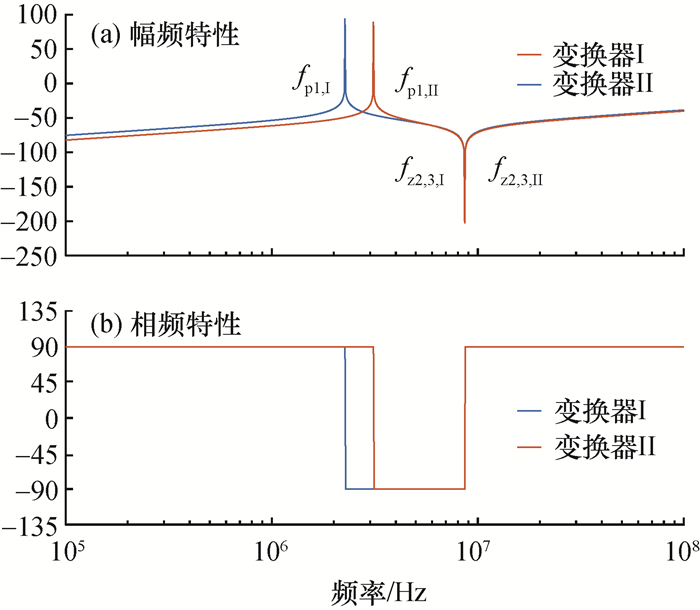图 17 (网络版彩图)全受控模式阻抗网络导纳波特图

 $\left\{ {\begin{array}{*{20}{l}} {{f_{{\rm{p1,I}}}} = 2.27{\kern 1pt} {\kern 1pt} {\kern 1pt} {\rm{MHz,}}}\\ {{f_{{\rm{p1,II}}}} = 3.12{\kern 1pt} {\kern 1pt} {\kern 1pt} {\rm{MHz}}{\rm{.}}} \end{array}} \right.$ (16)

 $\left\{ {\begin{array}{*{20}{l}} {{T_{\rm{I}}} = 0.44{\kern 1pt} {\kern 1pt} {\kern 1pt} \mu {\rm{s}},}\\ {{T_{{\rm{II}}}} = 0.32{\kern 1pt} {\kern 1pt} {\kern 1pt} \mu {\rm{s}}.} \end{array}} \right.$ (17)
4.3 系统参数对极点分布的影响

5 实验验证 5.1 实验平台简介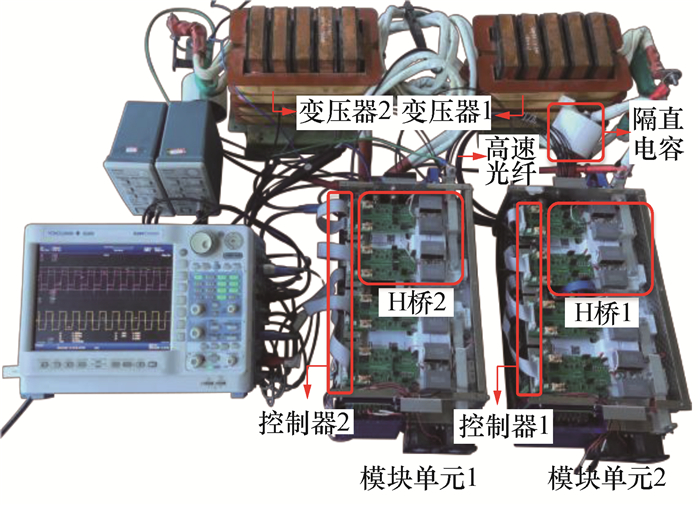图 18 (网络版彩图)实验平台

5.2 不控整流模式实验图 19 (网络版彩图)变换器Ⅰ负载80 kΩ时电压电流波形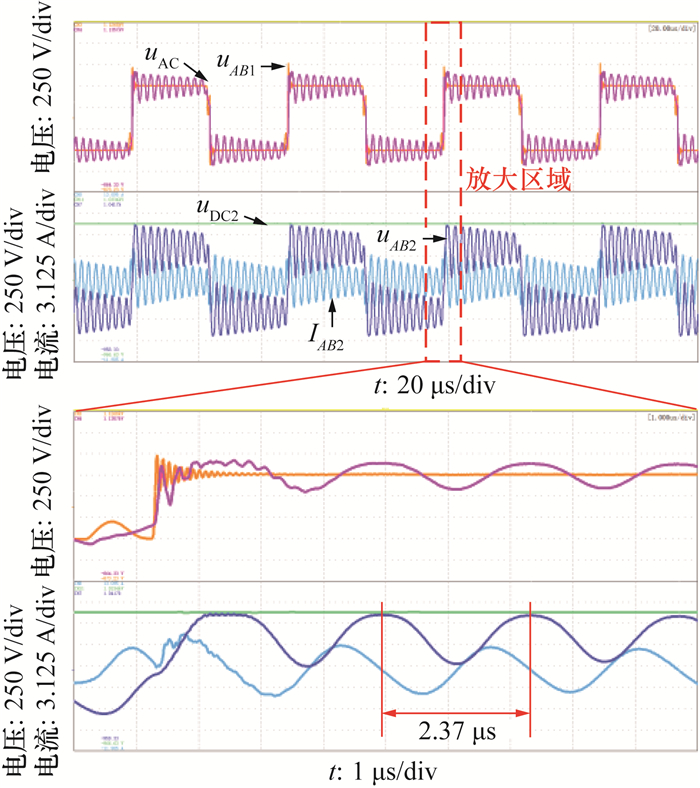图 20 (网络版彩图)变换器Ⅱ负载80 kΩ时电压电流波形

5.3 不控整流模式换流分析图 21 (网络版彩图)负载2 kΩ时电压电流波形

5.4 全受控模式下实验图 22 (网络版彩图)全受控模式80 kΩ负载波形

5.5 模式切换实验图 23 (网络版彩图)启动过程模式切换实验

t1时刻，模块单元1通过内部移相产生一个占空比逐渐增大的交流方波电压给单元2充电，在此阶段，高频交流母线电压uAC、模块2桥臂电压uAB2和电流iAB2有明显的振荡现象。在t2时刻，变换器由不控整流模式切换至全受控模式，由开关管结电容造成的振荡现象消失，同时，模块单元2的直流母线电压uDC2由446 V下降至400 V。

6 结论

  赵争鸣, 施博辰, 朱义诚. 对电力电子学的再认识——历史、现状及发展[J]. 电工技术学报, 2017, 32(12): 5-15. ZHAO Z M, SHI B C, ZHU Y C. Reconsideration on power electronics:The past, present and future[J]. Transactions of China Electrotechnical Society, 2017, 32(12): 5-15. (in Chinese)  冯高辉, 赵争鸣, 袁立强. 基于能量平衡的电能路由器综合控制技术[J]. 电工技术学报, 2017, 32(14): 34-44. FENG G H, ZHAO Z M, YUANL Q. Synthetical control technology of electric energy router based on energy blance relationship[J]. Transactions of China Electrotechnical Society, 2017, 32(14): 34-44. (in Chinese)  李子欣, 王平, 楚遵方, 等. 面向中高压智能配电网的电力电子变压器研究[J]. 电网技术, 2013, 37(9): 2592-2601. LI Z X, WANG P, CHU Z F, et al. Research on medium and high voltage smart distribution grid oriented power electronic transformer[J]. Power System Technology, 2013, 37(9): 2592-2601. (in Chinese)  段青, 徐嘉超, 盛万兴, 等. 基于级联高频变压器的多端口直流电能路由器[J]. 电网技术, 2019, 43(8): 2934-2941. DUAN Q, XU J C, SHENG W X, et al. Multi-port DC electric energy router based on cascaded high frequency transformer[J]. Power System Technology, 2019, 43(8): 2934-2941. (in Chinese)  ZUMEL P, FERNANDEZ C, LAZARO A. Overall analysis of a modular multi active bridge converter[C]//Proceedings of 2014 IEEE 15th Workshop on COMPEL. Santander, Spain: IEEE, 2014: 1093-5142.  LI K, ZHAO Z M, YUAN L Q, et al. Synergetic control of high-frequency-link based multi-port solid state transformer[C]//Proceedings of 2018 IEEE Energy Conversion Congress and Exposition (ECCE), Portland, USA: IEEE, 2018: 2329-3721.  张春朋, 李凯, 赵争鸣, 等.一种全隔离型多端口换流器: 201810366809.4. 2018-10-16. ZHANG C P, LI K, ZHAO Z M, et al. Full-isolation type multi-port converter. 201810366809. 4. 2018-10-16.(in Chinese)  李方正, 孙旭东, 黄立培, 等. 大容量变流器复杂形状直流母线的PEEC建模[J]. 清华大学学报(自然科学版), 2009, 49(8): 1089-1092. LI F Z, SUN X D, HUANG L P, et al. PEEC modeling of complex DC buses in high power converters[J]. Journal of Tsinghua University (Science and Technology), 2009, 49(8): 1089-1092. DOI:10.3321/j.issn:1000-0054.2009.08.003 (in Chinese)  于华龙, 赵争鸣, 袁立强, 等. 高压IGBT串联变换器直流母排设计与杂散参数分析[J]. 清华大学学报(自然科学版), 2014, 54(4): 540-545. YU H L, ZHAO Z M, YUAN L Q, et al. High-voltage IGBTs series converter bus bar design and stray parameter analysis[J]. Journal of Tsinghua University (Science and Technology), 2014, 54(4): 540-545. (in Chinese)  王旭东, 朱义诚, 赵争鸣, 等. 驱动回路参数对碳化硅MOSFET开关瞬态过程的影响[J]. 电工技术学报, 2017, 32(13): 23-30. WANG X D, ZHU Y C, ZHAO Z M, et al. Impact of gate-loop parameters on the switching behavior of SiC MOSFETs[J]. Transactions of China Electrotechnical Society, 2017, 32(13): 23-30. (in Chinese)  赵争鸣, 袁立强, 鲁挺. 电力电子系统电磁瞬态过程[M]. 北京: 清华大学出版社, 2017. ZHAO Z M, YUAN L Q, LU T. Electromagnetic transients of power electronics systems[M]. Beijing: Tsinghua University Press, 2017. (in Chinese)  CHEN W, LEE F C, JOVANOVIC M M, et al. A comparative study of a class of full bridge zero-voltage-switched PWM converters[C]//Proceedings of 1995 IEEE Applied Power Electronics Conference and Exposition (APEC). Dallas, USA: IEEE, 1995: 893-899.  阮新波. 脉宽调制DC/DC全桥变换器的软开关技术[M]. 北京: 科学出版社, 2012. RUAN X B. Soft switching technology of Full-bridge DC/DC converter based on pulse width modulation[M]. Beijing: Science Press, 2012. (in Chinese)  崔彬, 李欣阳, 薛芃, 等. 双主动全桥变换器的高频振荡影响因素[J]. 清华大学学报(自然科学版), 2020, 60(6): 530-536. CUI B, LI X Y, XUE P, et al. Factors influencing high frequency oscillations in dual active bridge converters[J]. Journal of Tsinghua University (Science and Technology), 2020, 60(6): 530-536. (in Chinese)  QIN Z, SHEN Z, BLAABJERG F. Modelling and analysis of the transformer current resonance in dual active bridge converters[C]//Proceedings of 2017 IEEE Energy Conversion Congress and Exposition (ECCE). Cincinnati, USA: IEEE, 2017: 4520-4524.  SUN J N, YUAN L Q, GU Q, et al. Startup strategy with constant peak transformer current for solid-state transformer in distribution network[J]. IEEE Transactions on Industry Application, 2019, 55(2): 1740-1751. DOI:10.1109/TIA.2018.2883012  MUSZNICKI P, CHRZAN P J, RUCINSKI M, et al. Adaptive estimation of the transformer stray capacitances for DC-DC converter modelling[J]. IET Power Electronics, 2016, 9(15): 2865-2870. DOI:10.1049/iet-pel.2016.0053  DALESSANDRO L, SILVEIRA C, Kolar J W. Self-capacitance of high-voltage transformers[J]. IEEE Transactions on Power Electronics, 2017, 22(5): 2081-2092.  MASSARINI A, KAZIMIERCZUK M K. Self capacitance of inductors[J]. IEEE Transactions on Power Electronics, 1997, 12(4): 671-676. DOI:10.1109/63.602562  CHEN K N, ZHAO Z M, YUAN L Q, et al. The impact of nonlinear junction capacitance on switching transient and it's modeling for SiC MOSFET[J]. IEEE Transactions on Electron Devices, 2015, 62(2): 333-338. DOI:10.1109/TED.2014.2362657  CUI B, XUE P, JIANG X H. Elimination of high frequency oscillation in dual active bridge converters by dv/dt optimization[J]. IEEE Access, 2019, 7: 55554-55564. DOI:10.1109/ACCESS.2019.2910597